### Excel FILTER Function## Excel FILTER Function

I really like Excel’s AutoFilter but I think the Excel FILTER function does the job better because it’s a live formula. And it’s an extraction, you don’t have to filter your data in place. This means it’s easy to have your results wherever you want them without having to bother with the Advanced Filter.

It’s a fairly new function so, as usual, the first thing is to check out whether you have it or not. Just click on an Excel cell, type-in “=FI” and see if you can spot FILTER in the pop-up list.

Here’s the data I want to filter, it’s a simple Excel list with 6 columns, A to F, with headings entered into the top row.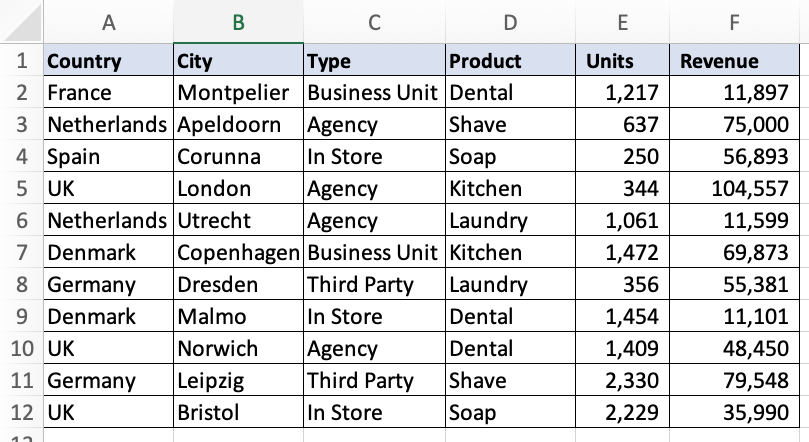## Basic Excel Filter Formula

And here’s the basic filter formula. I want to filter the list to just show the rows where I have “Agency” in column C.

=FILTER(A:F,C:C=”Agency”)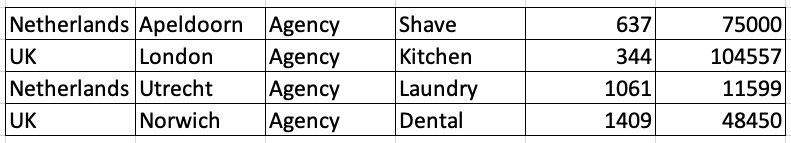## Sorting the Results

You can sort the results, if you want to, by wrapping the SORT function around the FILTER function, like this:

=SORT(FILTER(A:F,C:C=”Agency”))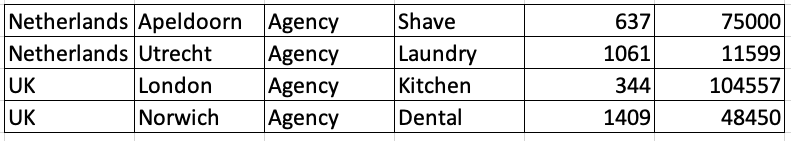And that sorts the results in alpha order on the first column. But you can change this by entering extra information into the sort. In this example, I’m sorting the results by the 6th column (that’s my last column) with the highest number listed first. So, that’s minus 1 for descending order. Here’s the formula:

=SORT(FILTER(A:F,C:C=”Agency”),6,-1)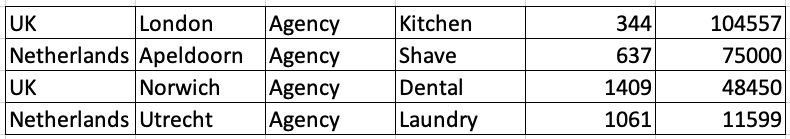## Filtering the Filter

Here’s the original filter formula again. You’ll see that the formula gives you all the columns from your data. You don’t always want that. To control the results you need to filter your filter.

=FILTER(A:F,C:C=”Agency”)To do this put your original filter inside another filter and use an array of values to declare which columns to display in the results. The array is a set of zeros or ones inside a pair of braces (squiggly brackets). A one means show the column, a zero hides the column. Here’s my formula; out of the 6 columns, I’m showing only the second, third and sixth columns:

=FILTER(FILTER(A:F,C:C=”Agency”),{0,1,1,0,0,1})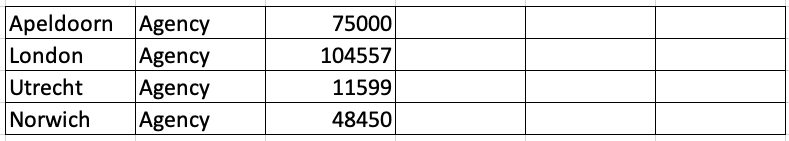## More Complex Excel Filter Formulas

You can create complex filters by multiplying different expressions in your filter. Don’t worry about the internal logic, just copy and paste an example and adapt it to your own needs. I always do!

In this example, include everything where you have “Agency” in column C and the value in column E is 1000 or more:

=FILTER(A:F,(C:C=”Agency”)*(E:E>=1000))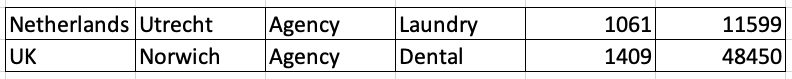In this one, include everything where the value in column E is between 250 and 1000:

=FILTER(A:F,(E:E>=250)*(E:E<=1000))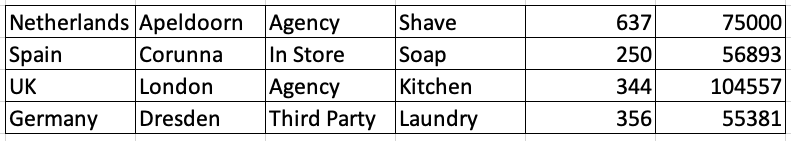In this one, include everything where the value in column E is between 250 and 1000, but not where the value in column C is “Agency”:

=FILTER(A:F,(E:E>=250)*(E:E<=1000)*NOT(C:C=”Agency”))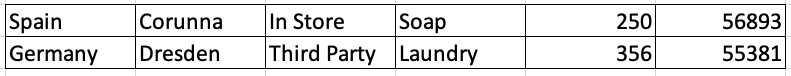Multiplication gives you a logical AND condition. If you want a logical OR, then use the plus sign. For example, this formula means include everything where the value in column C is either “Agency” or “In Store”:

=FILTER(A:F,(C:C=”Agency”)+(C:C=”In Store”))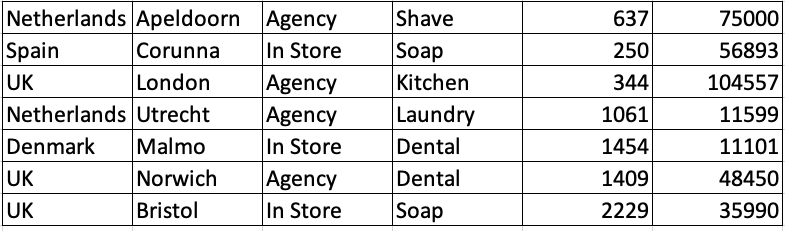That’s all for now, best of luck with your filters!The FILTER function is just one of the new type of Excel functions, Dynamic Array Functions that were released with Excel 365. To learn more about these revolutionary functions, come along on our dedicated Excel Dynamic Array Functions Course.

Call 020 7920 9500 now for details.